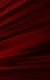# Super Simple Formula

As mentioned in about programming, computers ultimately just do simple math (like addition) and store and retrieve data, but they are able to accomplish many things with these few operations.

When programming, you'll often need to write simple formulas for the computer to calculate. For example, you would write `x-y` as a 'formula' for the difference between two numbers `x` and `y`.

We will learn how to get the computer to do some simple math for us. To get started, try solving this simple problem.

### Challenge

You are given two variables `a` and `b`. Write the formula for the sum of `a` and `b`.

Alternatively, you can try out Learneroo before signing up.

•#### Alger

it will become harder

•#### ciel

how do you know.

•#### Jolly

it does become much harder

•#### Jolly

im on arrays now

•#### Gaetano

is there a way to reset your progress?